# R S Aggarwal Solutions for Class 10 Maths Exercise 19A Chapter 19 Probability

These R S Aggarwal solutions for class 10 Maths are prepared by subject experts after thorough research. Solutions for all the questions are available in a simple language as students can learn and understand the entire concept easily. Chapter 19 exercise 19A is all about the probability of occurrence of an event. Download pdf of Class 10 Maths Chapter 19 R S Aggarwal Solutions and practice offline.

## Download PDF of R S Aggarwal Solutions for Class 10 Maths Chapter 19 Probability Exercise 19A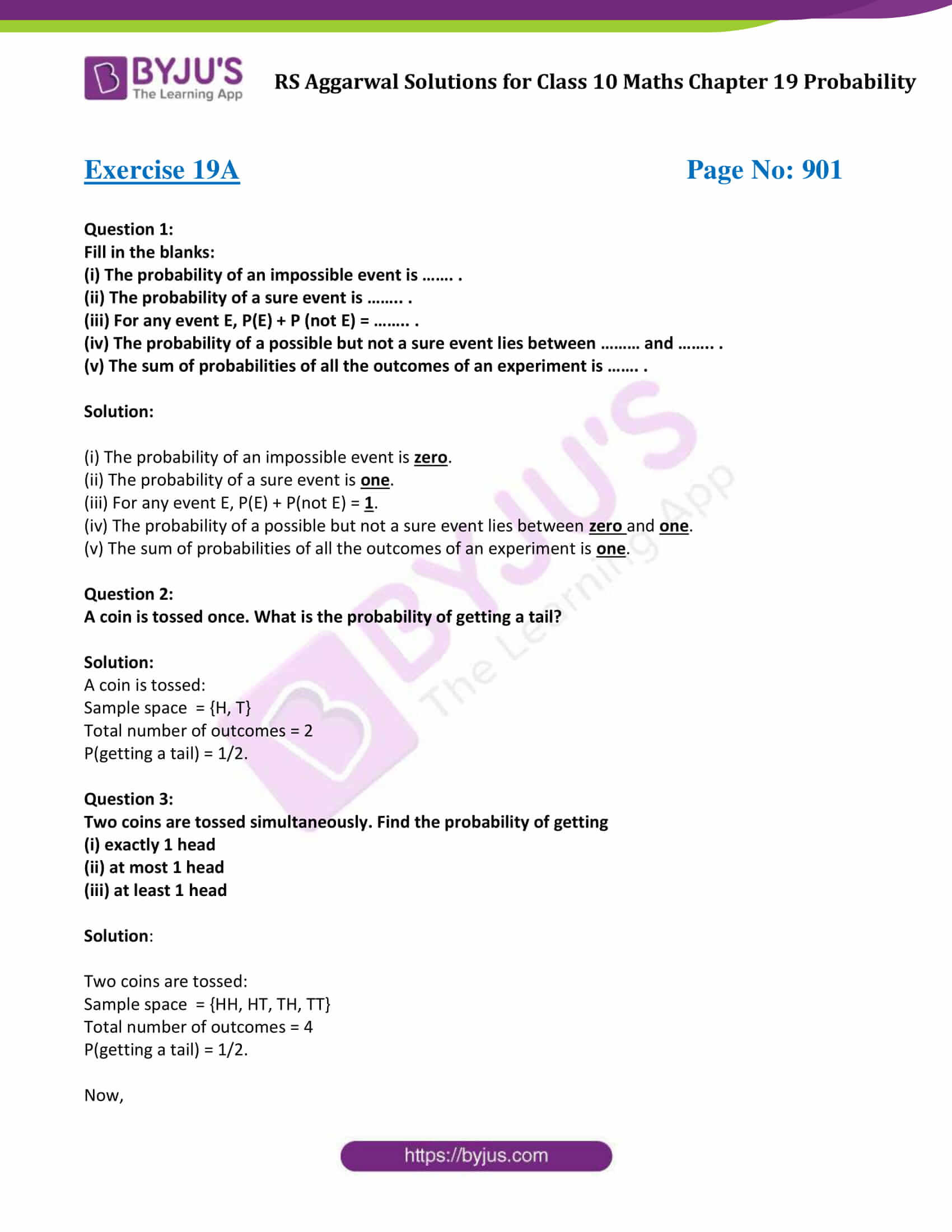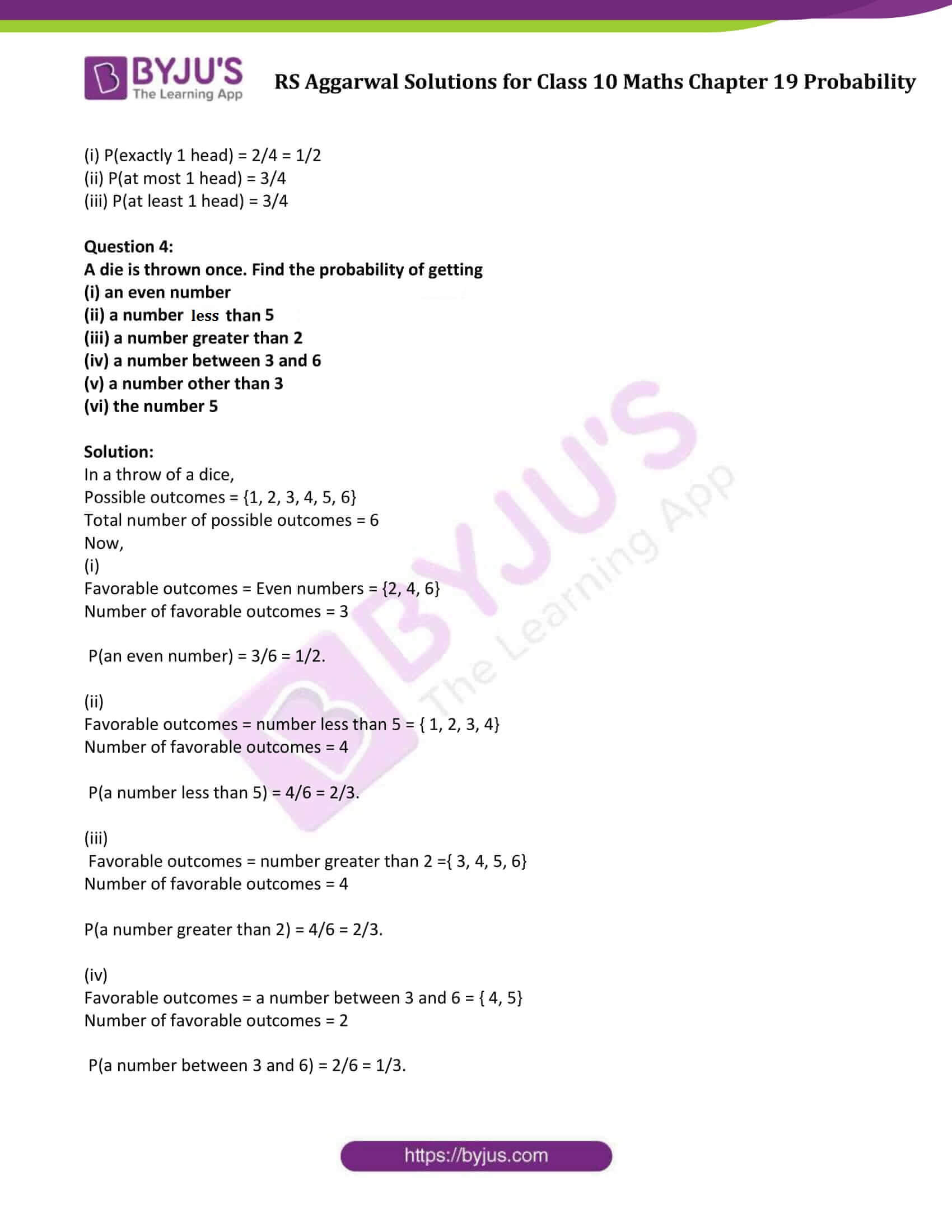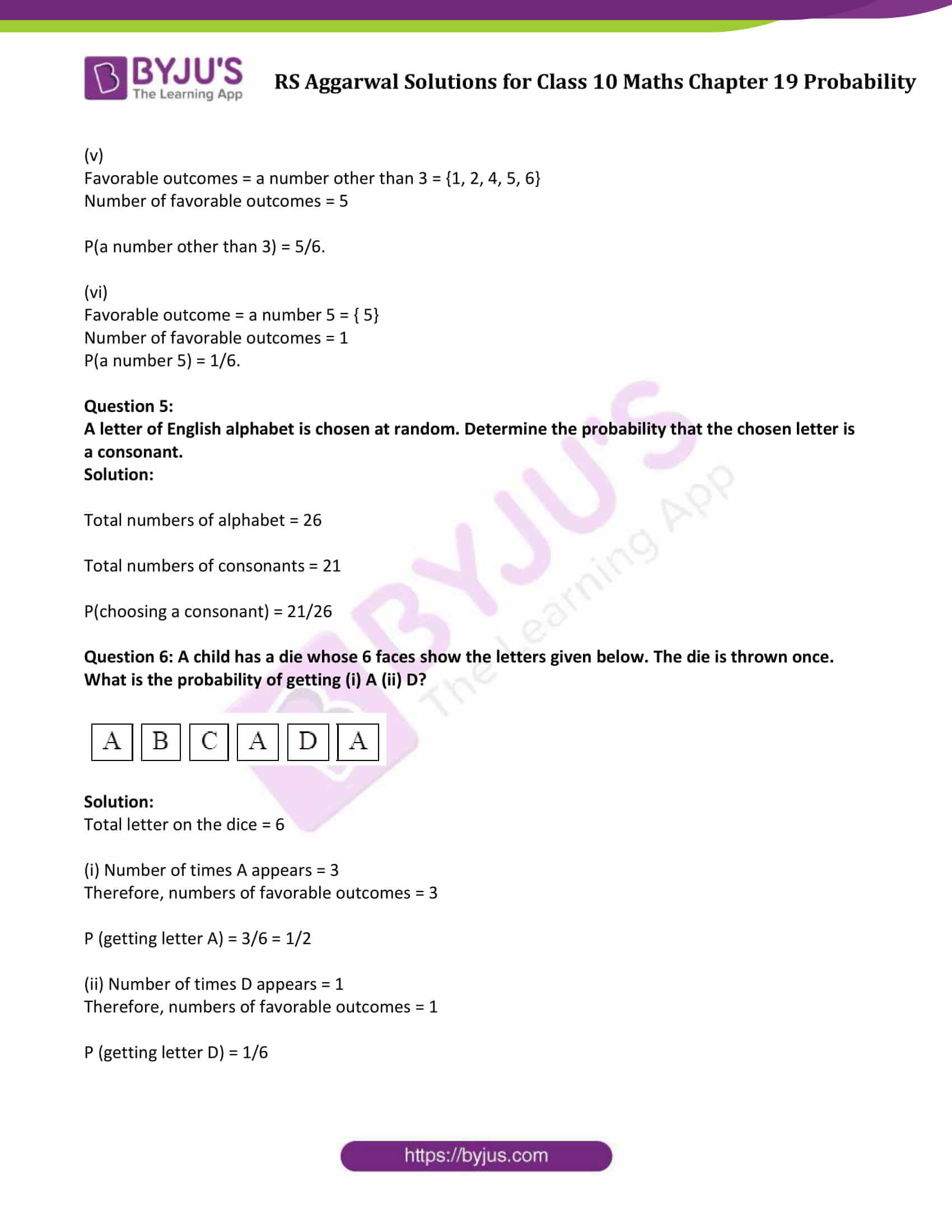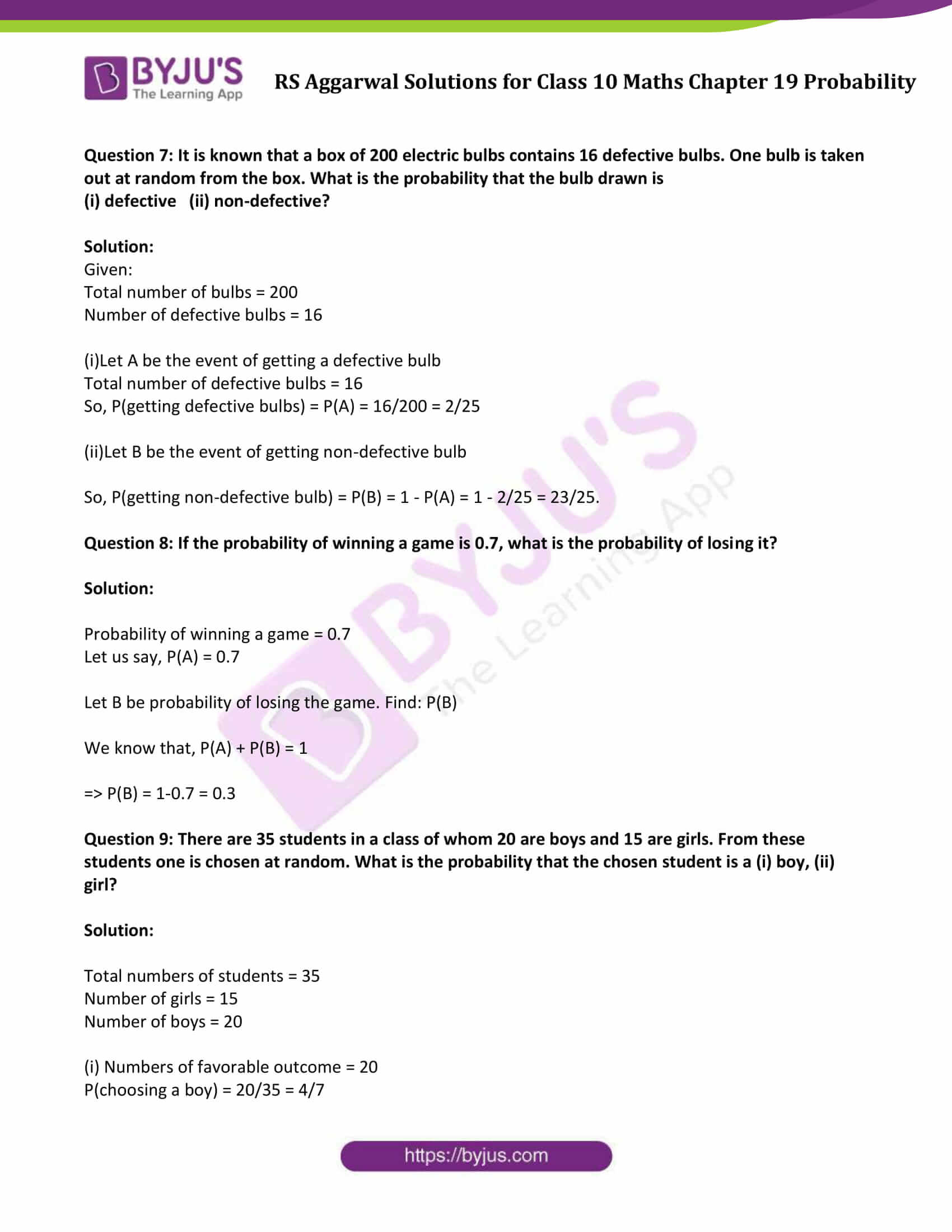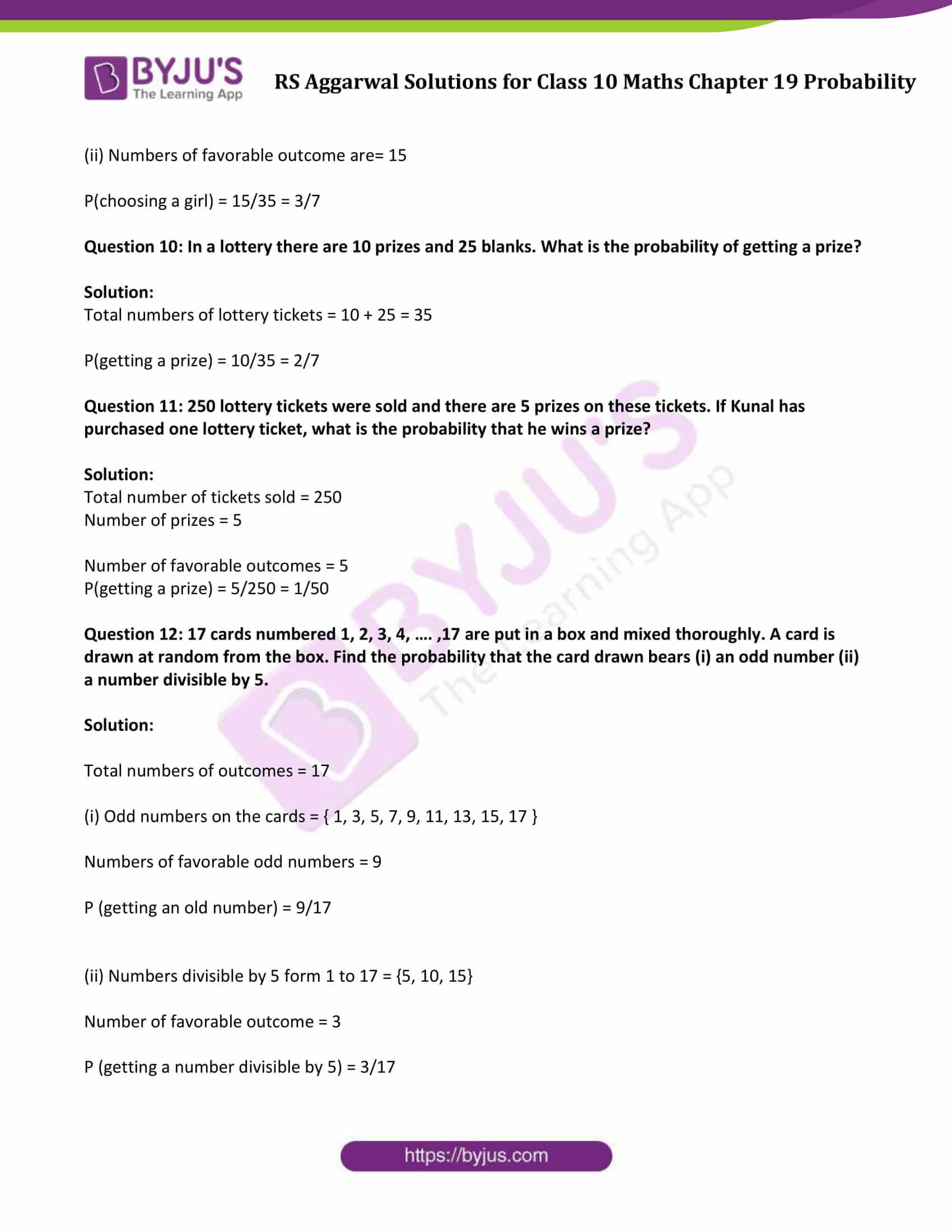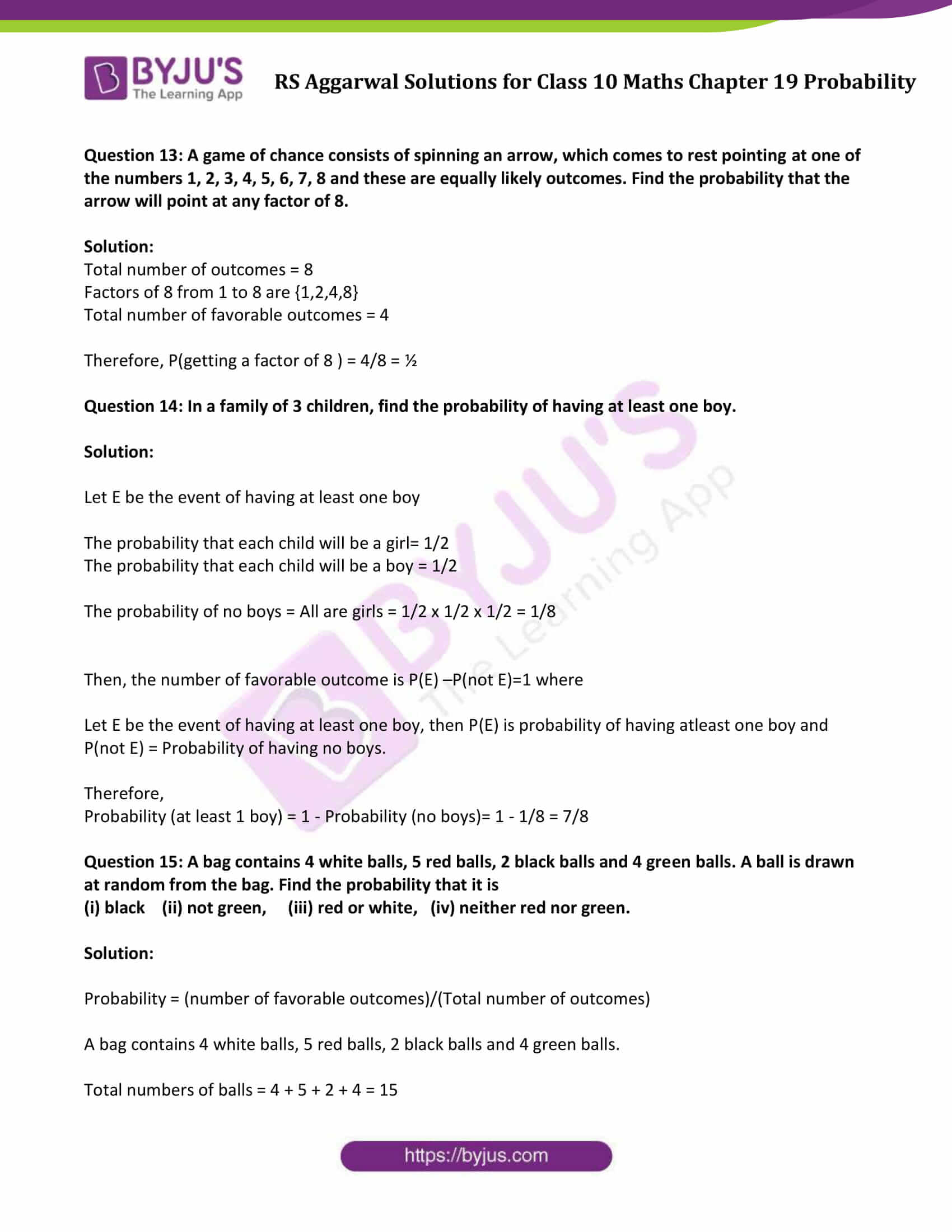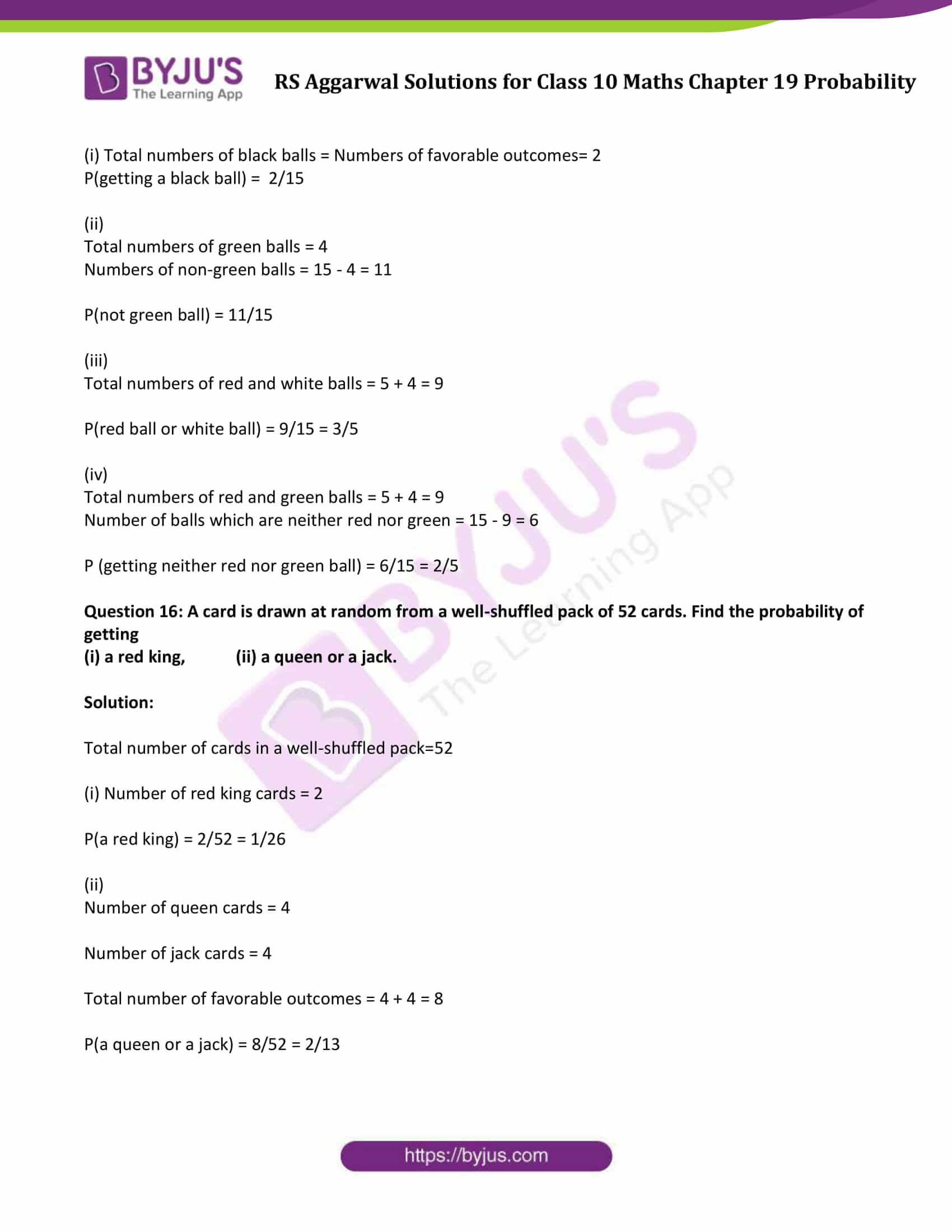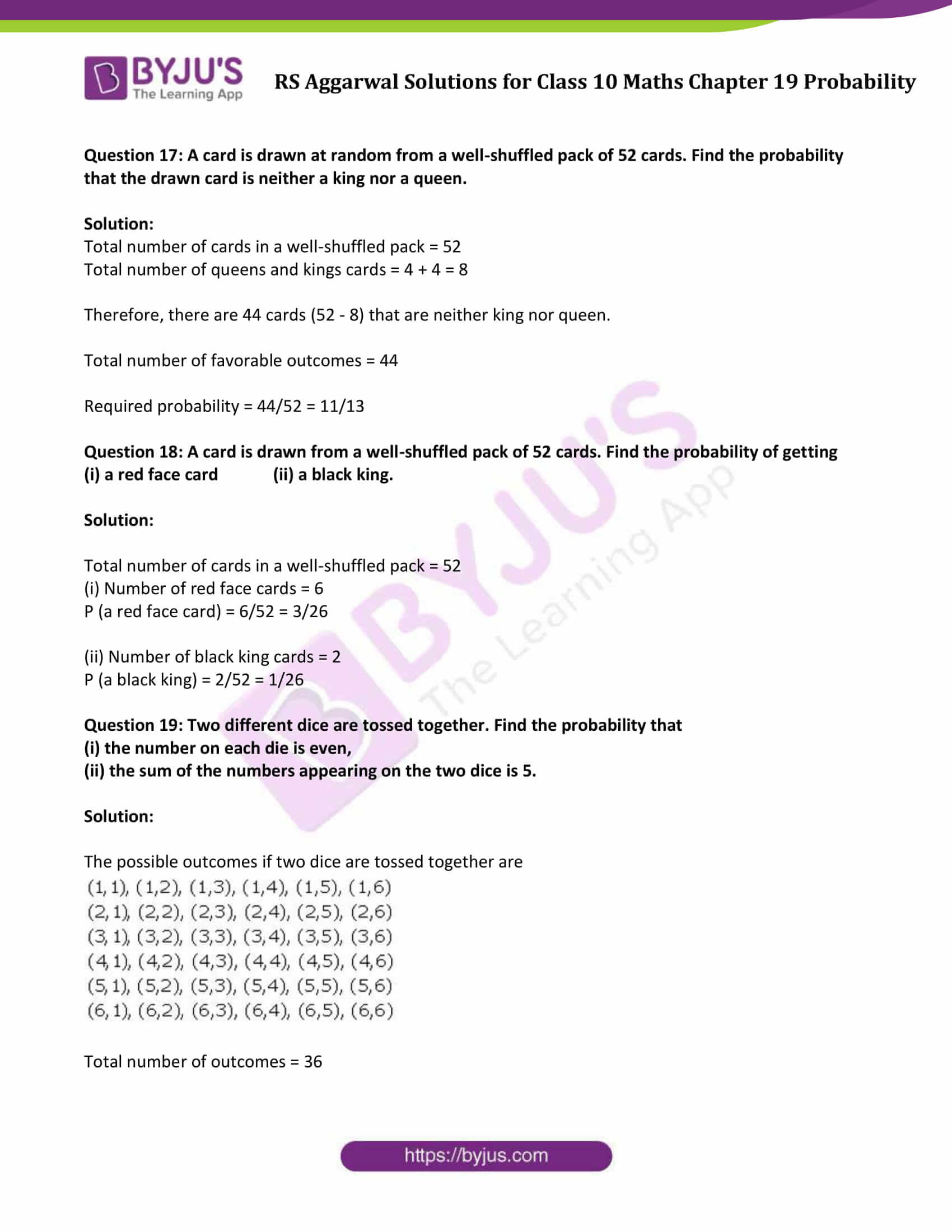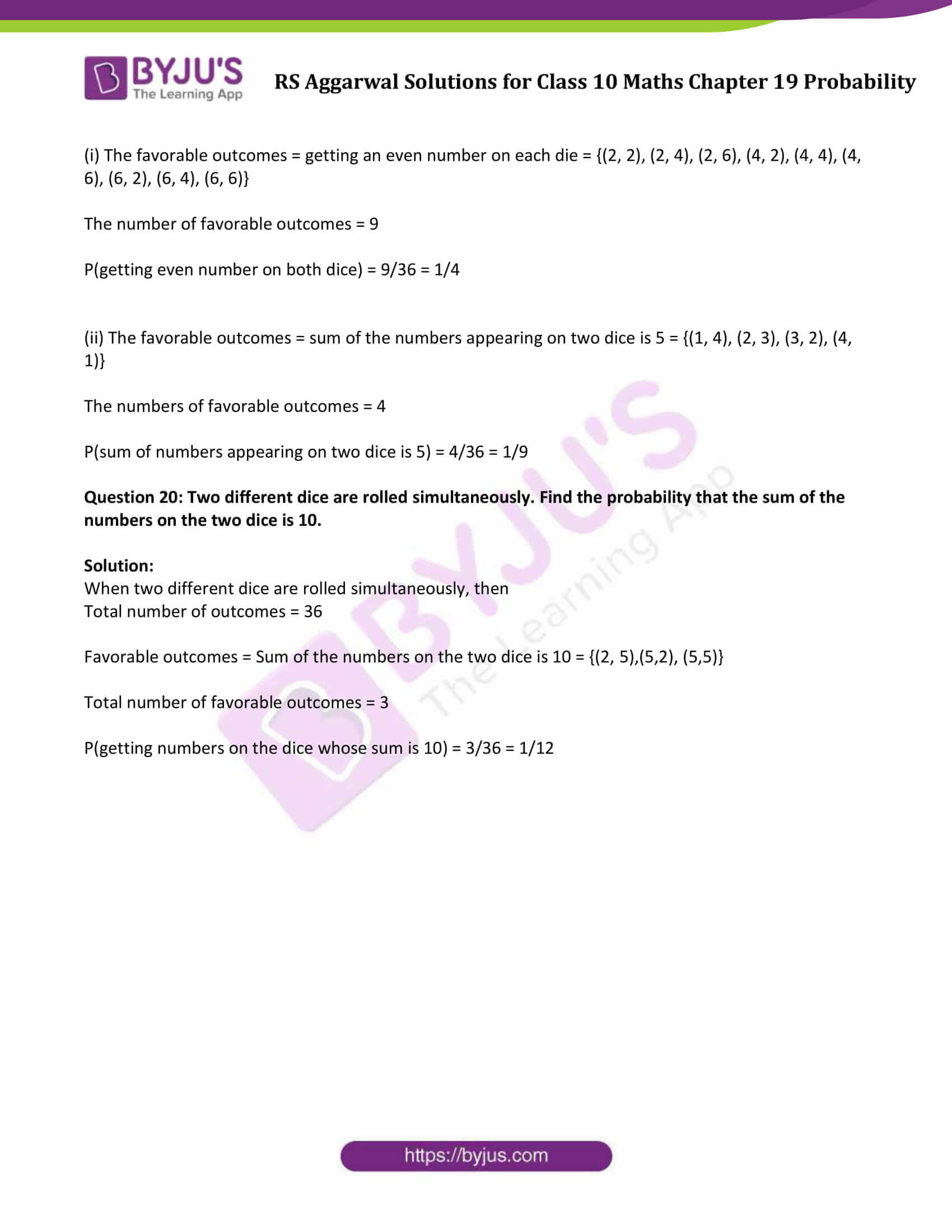### Access Solutions to Maths R S Aggarwal Class 10 Chapter 19 Probability Exercise 19A

Exercise 19A

Question 1:

Fill in the blanks:

(i) The probability of an impossible event is ……. .

(ii) The probability of a sure event is …….. .

(iii) For any event E, P(E) + P (not E) = …….. .

(iv) The probability of a possible but not a sure event lies between ……… and …….. .

(v) The sum of probabilities of all the outcomes of an experiment is ……. .

Solution:

(i) The probability of an impossible event is zero.

(ii) The probability of a sure event is one.

(iii) For any event E, P(E) + P(not E) = 1.

(iv) The probability of a possible but not a sure event lies between zero and one.

(v) The sum of probabilities of all the outcomes of an experiment is one.

Question 2:

A coin is tossed once. What is the probability of getting a tail?

Solution:

A coin is tossed:

Sample space = {H, T}

Total number of outcomes = 2

P(getting a tail) = 1/2.

Question 3:

Two coins are tossed simultaneously. Find the probability of getting

Solution:

Two coins are tossed:

Sample space = {HH, HT, TH, TT}

Total number of outcomes = 4

P(getting a tail) = 1/2.

Now,

(i) P(exactly 1 head) = 2/4 = 1/2

(ii) P(at most 1 head) = 3/4

(iii) P(at least 1 head) = 3/4

Question 4:

A die is thrown once. Find the probability of getting

(i) an even number

(ii) a number less than 5

(iii) a number greater than 2

(iv) a number between 3 and 6

(v) a number other than 3

(vi) the number 5

Solution:

In a throw of a dice,

Possible outcomes = {1, 2, 3, 4, 5, 6}

Total number of possible outcomes = 6

Now,

(i)

Favorable outcomes = Even numbers = {2, 4, 6}

Number of favorable outcomes = 3

P(an even number) = 3/6 = 1/2.

(ii)

Favorable outcomes = number less than 5 = { 1, 2, 3, 4}

Number of favorable outcomes = 4

P(a number less than 5) = 4/6 = 2/3.

(iii)

Favorable outcomes = number greater than 2 ={ 3, 4, 5, 6}

Number of favorable outcomes = 4

P(a number greater than 2) = 4/6 = 2/3.

(iv)

Favorable outcomes = a number between 3 and 6 = { 4, 5}

Number of favorable outcomes = 2

P(a number between 3 and 6) = 2/6 = 1/3.

(v)

Favorable outcomes = a number other than 3 = {1, 2, 4, 5, 6}

Number of favorable outcomes = 5

P(a number other than 3) = 5/6.

(vi)

Favorable outcome = a number 5 = { 5}

Number of favorable outcomes = 1

P(a number 5) = 1/6.

Question 5:

A letter of English alphabet is chosen at random. Determine the probability that the chosen letter is a consonant.

Solution:

Total numbers of alphabet = 26

Total numbers of consonants = 21

P(choosing a consonant) = 21/26

Question 6: A child has a die whose 6 faces show the letters given below. The die is thrown once. What is the probability of getting (i) A (ii) D?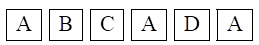Solution:

Total letter on the dice = 6

(i) Number of times A appears = 3

Therefore, numbers of favorable outcomes = 3

P (getting letter A) = 3/6 = 1/2

(ii) Number of times D appears = 1

Therefore, numbers of favorable outcomes = 1

P (getting letter D) = 1/6

Question 7: It is known that a box of 200 electric bulbs contains 16 defective bulbs. One bulb is taken out at random from the box. What is the probability that the bulb drawn is

(i) defective (ii) non-defective?

Solution:

Given:

Total number of bulbs = 200

Number of defective bulbs = 16

(i)Let A be the event of getting a defective bulb

Total number of defective bulbs = 16

So, P(getting defective bulbs) = P(A) = 16/200 = 2/25

(ii)Let B be the event of getting non-defective bulb

So, P(getting non-defective bulb) = P(B) = 1 – P(A) = 1 – 2/25 = 23/25.

Question 8: If the probability of winning a game is 0.7, what is the probability of losing it?

Solution:

Probability of winning a game = 0.7

Let us say, P(A) = 0.7

Let B be probability of losing the game. Find: P(B)

We know that, P(A) + P(B) = 1

=> P(B) = 1-0.7 = 0.3

Question 9: There are 35 students in a class of whom 20 are boys and 15 are girls. From these students one is chosen at random. What is the probability that the chosen student is a (i) boy, (ii) girl?

Solution:

Total numbers of students = 35

Number of girls = 15

Number of boys = 20

(i) Numbers of favorable outcome = 20

P(choosing a boy) = 20/35 = 4/7

(ii) Numbers of favorable outcome are= 15

P(choosing a girl) = 15/35 = 3/7

Question 10: In a lottery there are 10 prizes and 25 blanks. What is the probability of getting a prize?

Solution:

Total numbers of lottery tickets = 10 + 25 = 35

P(getting a prize) = 10/35 = 2/7

Question 11: 250 lottery tickets were sold and there are 5 prizes on these tickets. If Kunal has purchased one lottery ticket, what is the probability that he wins a prize?

Solution:

Total number of tickets sold = 250

Number of prizes = 5

Number of favorable outcomes = 5

P(getting a prize) = 5/250 = 1/50

Question 12: 17 cards numbered 1, 2, 3, 4, …. ,17 are put in a box and mixed thoroughly. A card is drawn at random from the box. Find the probability that the card drawn bears (i) an odd number (ii) a number divisible by 5.

Solution:

Total numbers of outcomes = 17

(i) Odd numbers on the cards = { 1, 3, 5, 7, 9, 11, 13, 15, 17 }

Numbers of favorable odd numbers = 9

P (getting an old number) = 9/17

(ii) Numbers divisible by 5 form 1 to 17 = {5, 10, 15}

Number of favorable outcome = 3

P (getting a number divisible by 5) = 3/17

Question 13: A game of chance consists of spinning an arrow, which comes to rest pointing at one of the numbers 1, 2, 3, 4, 5, 6, 7, 8 and these are equally likely outcomes. Find the probability that the arrow will point at any factor of 8.

Solution:

Total number of outcomes = 8

Factors of 8 from 1 to 8 are {1,2,4,8}

Total number of favorable outcomes = 4

Therefore, P(getting a factor of 8 ) = 4/8 = ½

Question 14: In a family of 3 children, find the probability of having at least one boy.

Solution:

Let E be the event of having at least one boy

The probability that each child will be a girl= 1/2

The probability that each child will be a boy = 1/2

The probability of no boys = All are girls = 1/2 x 1/2 x 1/2 = 1/8

Then, the number of favorable outcome is P(E) –P(not E)=1 where

Let E be the event of having at least one boy, then P(E) is probability of having atleast one boy and

P(not E) = Probability of having no boys.

Therefore,

Probability (at least 1 boy) = 1 – Probability (no boys)= 1 – 1/8 = 7/8

Question 15: A bag contains 4 white balls, 5 red balls, 2 black balls and 4 green balls. A ball is drawn at random from the bag. Find the probability that it is

(i) black (ii) not green, (iii) red or white, (iv) neither red nor green.

Solution:

Probability = (number of favorable outcomes)/(Total number of outcomes)

A bag contains 4 white balls, 5 red balls, 2 black balls and 4 green balls.

Total numbers of balls = 4 + 5 + 2 + 4 = 15

(i) Total numbers of black balls = Numbers of favorable outcomes= 2

P(getting a black ball) = 2/15

(ii)

Total numbers of green balls = 4

Numbers of non-green balls = 15 – 4 = 11

P(not green ball) = 11/15

(iii)

Total numbers of red and white balls = 5 + 4 = 9

P(red ball or white ball) = 9/15 = 3/5

(iv)

Total numbers of red and green balls = 5 + 4 = 9

Number of balls which are neither red nor green = 15 – 9 = 6

P (getting neither red nor green ball) = 6/15 = 2/5

Question 16: A card is drawn at random from a well-shuffled pack of 52 cards. Find the probability of getting

(i) a red king, (ii) a queen or a jack.

Solution:

Total number of cards in a well-shuffled pack=52

(i) Number of red king cards = 2

P(a red king) = 2/52 = 1/26

(ii)

Number of queen cards = 4

Number of jack cards = 4

Total number of favorable outcomes = 4 + 4 = 8

P(a queen or a jack) = 8/52 = 2/13

Question 17: A card is drawn at random from a well-shuffled pack of 52 cards. Find the probability that the drawn card is neither a king nor a queen.

Solution:

Total number of cards in a well-shuffled pack = 52

Total number of queens and kings cards = 4 + 4 = 8

Therefore, there are 44 cards (52 – 8) that are neither king nor queen.

Total number of favorable outcomes = 44

Required probability = 44/52 = 11/13

Question 18: A card is drawn from a well-shuffled pack of 52 cards. Find the probability of getting

(i) a red face card (ii) a black king.

Solution:

Total number of cards in a well-shuffled pack = 52

(i) Number of red face cards = 6

P (a red face card) = 6/52 = 3/26

(ii) Number of black king cards = 2

P (a black king) = 2/52 = 1/26

Question 19: Two different dice are tossed together. Find the probability that

(i) the number on each die is even,

(ii) the sum of the numbers appearing on the two dice is 5.

Solution:

The possible outcomes if two dice are tossed together are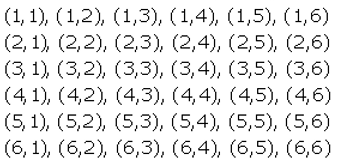Total number of outcomes = 36

(i) The favorable outcomes = getting an even number on each die = {(2, 2), (2, 4), (2, 6), (4, 2), (4, 4), (4, 6), (6, 2), (6, 4), (6, 6)}

The number of favorable outcomes = 9

P(getting even number on both dice) = 9/36 = 1/4

(ii) The favorable outcomes = sum of the numbers appearing on two dice is 5 = {(1, 4), (2, 3), (3, 2), (4, 1)}

The numbers of favorable outcomes = 4

P(sum of numbers appearing on two dice is 5) = 4/36 = 1/9

Question 20: Two different dice are rolled simultaneously. Find the probability that the sum of the numbers on the two dice is 10.

Solution:

When two different dice are rolled simultaneously, then

Total number of outcomes = 36

Favorable outcomes = Sum of the numbers on the two dice is 10 = {(2, 5),(5,2), (5,5)}

Total number of favorable outcomes = 3

P(getting numbers on the dice whose sum is 10) = 3/36 = 1/12

## Access other exercise solutions of Class 10 Maths Chapter 19 Probability

Exercise 19B Solutions

## R S Aggarwal Solutions for Class 10 Maths Chapter 19 Probability Exercise 19A Topics:

Class 10 Maths Chapter 19 Probability Exercise 19A is based on the following topics and subtopics:

• Random Experiment
• Possible outcomes in various experiments
• Equally likely terms
• Probability of occurrence of an event
• Sure events and impossible events
• Complementary events
• Word Problems in real-life situations